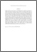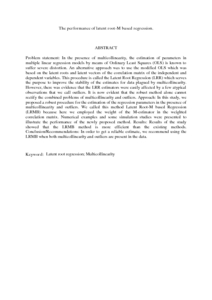# The performance of latent root-M based regression.

## Citation

Midi, Habshah and Lau, Ung Hua (2009) The performance of latent root-M based regression. Journal of Mathematics and Statistics, 5 (1). pp. 1-9. ISSN 1549-3644

## Abstract

Problem statement: In the presence of multicollinearity, the estimation of parameters in multiple linear regression models by means of Ordinary Least Squares (OLS) is known to suffer severe distortion. An alternative approach was to use the modified OLS which was based on the latent roots and latent vectors of the correlation matrix of the independent and dependent variables. This procedure is called the Latent Root Regression (LRR) which serves the purpose to improve the stability of the estimates for data plagued by multicollinearity. However, there was evidence that the LRR estimators were easily affected by a few atypical observations that we call outliers. It is now evident that the robust method alone cannot rectify the combined problems of multicollinearity and outliers. Approach: In this study, we proposed a robust procedure for the estimation of the regression parameters in the presence of multicollinearity and outliers. We called this method Latent Root-M based Regression (LRMB) because here we employed the weight of the M-estimator in the weighted correlation matrix. Numerical examples and some simulation studies were presented to illustrate the performance of the newly proposed method. Results: Results of the study showed that the LRMB method is more efficient than the existing methods. Conclusion/Recommendations: In order to get a reliable estimate, we recommend using the LRMB when both multicollinearity and outliers are present in the data.Preview
PDF (Abstract)
The performance of latent root.pdfView Item